Question

Approximating the eye as a single thin lens 2.70 cmcm from the retina, find the focal length of the eye when it is focused on an object at a distance of 265 cmcm

1.0.37 cm

Explanation:

The image is formed on the retina which is at a constant distance of 2.70 cm to the lens. Therefore, image distance = 2.70 cm.

The object is at a distant of 265 cm to the lens of the eye.

From lens formula,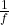=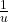+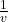where: f is the focal length, u is the object distance and v is the image distance.

Thus, u = 265.00 cm and v = 2.70 cm.=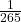+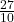=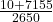=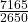⇒ f == 0.37

The focal length of the eye is 0.37 cm.